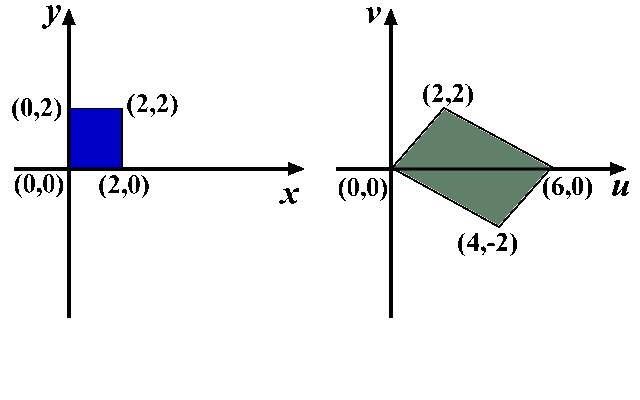# Change of Variables in Multiple Integrals

## Introduction

Substitution (or change of variables) is a powerful technique for evaluating integrals in single variable calculus. An equivalent transformation is available for dealing with multiple integrals. The idea is to replace the original variables of integration by the new set of variables. This way the integrand is changed as well as the bounds for integration. If we are lucky enough to find a convenient change of variables we can significantly simplify the integrand or the bounds.

## Change of variables formula

Two dimensional pictures are the easiest to draw so we will start with functions of two variables. Our first task is to get familiar with transformations of two dimensional regions.

### Transformations in $$\mathbb R^2$$

Assume that $$S$$ is a region in $$\mathbb R^2$$. We want to study the ways in which this region can be transformed to another region $$T$$.

This is easiest to explain by considering an example. Let $$S=[0,2]\times[0,2]$$. Consider the functions $$u:S\to \mathbb R$$ and $$v:S\to\mathbb R$$ defined in the following way: \begin{eqnarray*} u(x,y)&=&x+2y\\ v(x,y)&=&x-y.\end{eqnarray*} To every point $$(x,y)\in S$$ ($$S$$ is painted blue in the diagram on the left) we can assign a new green point with coordinates $$(u(x,y),v(x,y))$$. This way we obtain a green region $$T$$.The mapping $$(x,y)\mapsto (u(x,y),v(x,y))$$ is one-to-one and onto, hence a bijection (you may want to review these terms in the section Functions). We can also write the inverse transformation, that maps each point $$(u,v)\in T$$ to the point $$(x(u,v),y(u,v))$$ in the following way: \begin{eqnarray*} x(u,v)&=&\frac{u+2v}3 \\ y(u,v)&=&\frac{u-v}3. \end{eqnarray*}

### Change of variables in double integrals

Assume that $$S\subseteq \mathbb R^2$$ is a region in the plane. Let $$T\subseteq\mathbb R^2$$ be another region and assume that there are continuously differentiable functions $$X:T\to\mathbb R$$ and $$Y:T\to\mathbb R$$, such that the mapping $$\Phi(u,v)= (X(u,v),Y(u,v))$$ is a bijection between $$T$$ and $$S$$.

The Jacobian of the mapping $$\Phi$$ is defined as $\frac{\partial(X,Y)}{\partial(u,v)}=\det\left|\begin{array}{cc} \frac{\partial X}{\partial u}& \frac{\partial X}{\partial v}\\ \frac{\partial Y}{\partial u}& \frac{\partial Y}{\partial v}\end{array}\right|.$

Theorem (Change of variables in double integrals)

Assume that $$S$$ and $$T$$ are domains in $$\mathbb R^2$$ and that there are two continuously differentiable functions $$X,Y:T\to \mathbb R$$ such that $$\Phi: T\to S$$ defined by $$\Phi(u,v)=(X(u,v),Y(u,v))$$ is a bijection whose Jacobian is never $$0$$. For each continuous bounded $$f:S\to\mathbb R$$ the following equality holds: $\iint_S f(x,y)\,dxdy=\iint_T f(X(u,v),Y(u,v)) \cdot \left|\frac{\partial(X,Y)}{\partial(u,v)}\right|\,dudv.$

Example 1.

Using the substitution $$u=2x+3y$$, $$v=x-3y$$, find the value of the integral $\iint_D e^{2x+3y}\cdot \cos(x-3y)\,dxdy,$ where $$D$$ is the region bounded by the parallelogram with vertices $$(0,0)$$, $$\left(1,\frac13\right)$$, $$\left(\frac43,\frac19\right)$$, and $$\left(\frac13,-\frac29\right)$$.

### Change of variables in triple integrals

Assume that $$S, T\subseteq \mathbb R^3$$ are two regions in space. Assume that there are continuously differentiable functions $$X:T\to\mathbb R$$, $$Y:T\to\mathbb R$$, and $$Z:T\to\mathbb R$$, such that the mapping $$\Phi:T\to S$$ defined as $$\Phi(u,v,w)= (X(u,v,w),Y(u,v,w),Z(u,v,w))$$ is a bijection.

The Jacobian of the mapping $$\Phi$$ is defined as $\frac{\partial(X,Y,Z)}{\partial(u,v,w)}=\det\left|\begin{array}{ccc} \frac{\partial X}{\partial u}& \frac{\partial X}{\partial v}& \frac{\partial X}{\partial w}\\ \frac{\partial Y}{\partial u}& \frac{\partial Y}{\partial v}& \frac{\partial Y}{\partial w} \\ \frac{\partial Z}{\partial u}& \frac{\partial Z}{\partial v}& \frac{\partial Z}{\partial w}\end{array}\right|.$

Theorem (Change of variables in triple integrals)

Assume that $$S$$ and $$T$$ are domains in $$\mathbb R^2$$ and that there are three continuously differentiable functions $$X,Y,Z:T\to \mathbb R$$ such that $$\Phi: T\to S$$ defined by $$\Phi(u,v,w)=(X(u,v,w),Y(u,v,w),Z(u,v,w))$$ is a bijection whose Jacobian is never $$0$$. For each continuous bounded function $$f:S\to\mathbb R$$ the following equality holds: $\iiint_S f(x,y,z)\,dxdydz=\iiint_T f(X(u,v,w),Y(u,v,w),Z(u,v,w)) \cdot \left|\frac{\partial(X,Y,Z)}{\partial(u,v,w)}\right|\,dudvdw.$

## Polar, cylindrical, and spherical substitutions

We will now study very important substitutions that are used to simplify integrations over circular, spherical, cylindrical, and elliptical domains. One of them is applicable to double integral and is called polar change of variables and the other two, cylindrical and spherical, are used in triple integralds.

### Polar substitution

The following change of variables is called the polar substitution: \begin{eqnarray*} x&=&r\cos\theta\\ y&=&r\sin \theta. \end{eqnarray*} The Jacobian for the polar substitution is equal to: $\frac{\partial(x,y)}{\partial(r,\theta)}=\det\left|\begin{array}{cc} \cos\theta&-r\sin\theta\\ \sin\theta&r\cos\theta\end{array}\right|=r\cos^2\theta+r\sin^2\theta=r.$

The variables $$r$$ and $$\theta$$ have the geometric meaning in the $$xy$$-coordinate system. The distance between $$(x,y)$$ and the origin is precisely $$r$$, while $$\theta$$ is the angles between the $$x$$-axis and the line connecting $$(x,y)$$ with $$(0,0)$$.

Example 2.

Evaluate the integral $\iint_D \cos\left(x^2+y^2\right)\,dxdy,$ where $$D$$ is the disc of radius $$3$$ centered at the origin.

When dealing with ellipses it is very common to use the modified polar substitution. If the equation of the ellipse is $$\frac{x^2}{a^2}+\frac{y^2}{b^2}=1$$, the following substitution is used to describe its interior: \begin{eqnarray*} x&=&ar\cos\theta\\ y&=&br\sin\theta\\ 0\leq&r&\leq 1\\ 0\leq&\theta&\leq 2\pi. \end{eqnarray*}

Example 3.

Let $$a$$ and $$b$$ be two positive real numbers. Find the area of the region enclosed by the ellipse with the equation $$\frac{x^2}{a^2}+\frac{y^2}{b^2}=1$$.

### Cylindrical substitution

In cylindrical substitution the original variables $$(x,y,z)$$ are replaced by $$(r,\theta, z)$$ using the following equations: \begin{eqnarray*} x&=&r\cos\theta\\ y&=&r\sin\theta\\ z&=&z. \end{eqnarray*} Again we can find the Jacobian by calculating the appropriate determinant: $\frac{\partial(x,y,z)}{\partial(r,\theta,z)}=\det\left|\begin{array}{ccc} \cos\theta &-r\sin\theta &0\\ \sin\theta&r\cos\theta&0\\ 0&0&1\end{array}\right|=r .$

Example 4.

Determine the value of the integral $\iiint_D e^{x^2+y^2}\,dV$ where $$D$$ is the the region in bounded by the planes $$y=0$$, $$z=0$$, $$y=x$$, and the paraboloid $$z=4-x^2-y^2$$.

### Spherical substitution

Spherical substitution means replacing the original variables $$(x,y,z)$$ by the variables $$(\rho,\theta, \phi)$$, where $$\rho$$ is the distance of the points $$(x,y,z)$$ from the origin $$(0,0,0)$$; $$\theta$$ is the angle that the line connecting $$(0,0,0)$$ and $$(x,y,0)$$ forms with the $$x$$-axis, and $$\phi$$ is the angle between the $$z$$-axis and the line connecting $$(x,y,z)$$ with $$(0,0,0)$$. Mathematically, the equations are: \begin{eqnarray*} x&=&\rho\cos\theta\sin\phi\\ y&=&\rho\sin\theta\sin\phi\\ z&=&\rho\cos\phi. \end{eqnarray*} We can find the Jacobian by calculating the appropriate determinant: \begin{eqnarray*} \frac{\partial(x,y,z)}{\partial(\rho,\theta,\phi)}&=&\det\left|\begin{array}{ccc} \cos\theta\sin\phi &-\rho\sin\theta\sin\phi &\rho\cos\theta\cos\phi\\ \sin\theta\sin\phi&\rho\cos\theta\sin\phi&\rho\sin\theta\cos\phi\\ \cos\phi&0&-\rho\sin\phi\end{array}\right| \\ &=&-\rho^2\cos^2\theta\sin^3\phi-\rho^2\sin^2\theta\sin\phi\cos^2\phi-\rho^2\cos^2\theta\sin\phi\cos^2\phi-\rho^2\sin^2\theta\sin^3\phi \\&=&-\rho^2\sin^3\phi-\rho^2\sin\phi\cos^2\phi=-\rho^2\sin\phi .\end{eqnarray*} Since in evaluation of the integral we are using the absolute value of the Jacobian, and $$\phi\in\left(0,\frac{\pi}2\right)$$ it is sufficient and more convenient to remember that $\left|\frac{\partial(x,y,z)}{\partial(\rho,\theta,\phi)}\right|=\rho^2\sin\phi.$

Example 5.

Determine the value of the integral $\iiint_D e^{\sqrt{x^2+y^2+z^2}}\,dV$ where $$D$$ is the the region in bounded by the planes $$y=0$$, $$z=0$$, $$y=x$$, and the sphere $$x^2+y^2+z^2=9$$.

2005-2021 IMOmath.com | imomath"at"gmail.com | Math rendered by MathJax